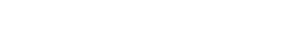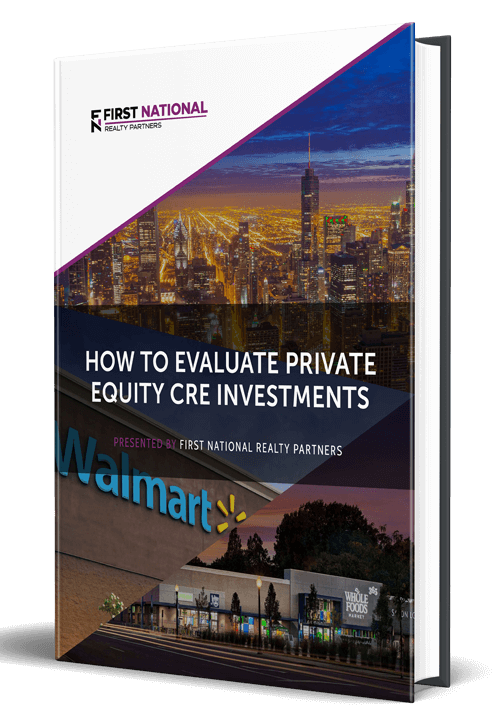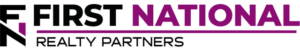# Gross Income Multiplier in Commercial Real Estate Valuation

### Key Takeaways

• The Gross Income Multiplier is a metric used to value a commercial property.
• It is calculated as the sale price of the property divided by the gross income it produces.
• As a general rule, a gross income multiplier of 4 to 7 is considered to be “good.” But, it is important to note that “good” is highly dependent upon a number of factors including market conditions and individual investor preferences.
• The major advantage of using the Gross Income Multiplier to value a property is that it is very simple and can be done quickly. In addition, it can be helpful to compare properties of different types and sizes.
• The major downside is that it does not take into account a property’s operating expenses, which is a major determinant of value.
• For this reason, Gross Income Multiplier is often used in conjunction with other valuation metrics like Gross Rent Multiplier and Net Operating Income.
• Because valuing a property as part of a purchase or sale can be complex, it may be helpful for individual investors to work with an expert, like a private equity firm, to complete a transaction.

Perhaps the most critical element in determining whether or not a commercial real estate investment makes a profit is the property’s valuation, on both the buy side and sell side. On the buy side, investors want to pay as little as possible and, on the sell side, they want to maximize the sales price. But, it can be tricky to value a property – there are many different ways to do it and many assumptions that must be made.

In this article, we are going to describe one valuation approach via a commonly used – the Gross Income Income Multiplier. We will describe what it is, how it is calculated, why it matters, and the pros and cons of using it to value a property.

At First National Realty Partners, we specialize in the acquisition and management of grocery store anchored retail centers. As part of the purchase (and sale) due diligence process, we look at a number of valuation metrics – including the Gross Income Multiplier. If you are an accredited investor and would like to learn more about our current investment offerings, click here.

## What is the Gross Income Multiplier?

The Gross Income Multiplier is a metric used to value commercial real estate investment properties. It is used by buyers and sellers alike to calculate an approximate purchase/sale price and to compare the sales price of one property to another, relative to the amount of income it produces.

### How Is The Gross Income Multiplier Calculated?

The formula used to calculate the Gross Income Multiplier is simple, it is calculated as a commercial property’s sales price divided by its gross annual income. Let’s discuss each of these components individually.

While the sales price seems fairly self-explanatory, a concrete number may not always be known at the time. As a proxy, purchasers could use the asking price for the property. Or, sellers could use their planned sales price. In either event, investors may want to run a number of different scenarios for sales price to see how the Gross Income Multiplier changes.

Gross Rental Income is simply the total income produced by a property (rent + other sources) in a given year. This information can be obtained from looking at a combination of the rent roll, income statement, and/or property leases.

### What Does The Gross Income Multiplier Mean?

The result of the Gross Income Multiplier calculation is kind of like the price to earnings ratio for a share of stock.  It represents the amount of income produced relative to the sales price.  Or, some investors also use it to determine how long it would take to pay off a property using gross income.  So, a lower GRM means that it would be paid off faster.

For example, a property with a GIM of 5 would be paid off faster than a property with a GIM of 9.

### Gross Income Multiplier Example

To illustrate how the Gross Income Multiplier works, an example is helpful.

Suppose that an investor is considering the purchase of a property that has an asking price of \$5,000,000 (the sales price) and annual gross income of \$350,000.

In this scenario, the Gross Income Multiplier would be \$5,000,000 divided by \$350,000 or 14.2.

But, this calculation may be overly simplistic. To address this issue, there are two variations of Gross Income Multiplier that investors should be aware of.

### Potential Gross Income Multiplier

Properties are not always completely full or producing income at their maximum potential. Investors could look at these properties and see an opportunity to increase occupancy/income through better management or more aggressive marketing. In this scenario, an investor would want to calculate the Potential Gross Income Multiplier.

As the name suggests, the Potential Gross Income Multiplier takes into account the total potential income that a property could earn. As such, the formula used to calculate it is: Sales Price / Total Potential Rental Income.  Adding the extra potential income will likely reduce the Gross Income Multiplier, shortening the time to pay for the property from income.

### Effective Gross Income Multiplier

The other variation is the “Effective” Gross Income Multiplier which uses a monthly income figure instead of annual.  Doing so may provide some additional precision around the income multiplier calculation.

## What is a Good Gross Income Multiplier?

As a general rule, a “good” Gross Income Multiplier is somewhere in the range of 4 to 7, with the lower end of the range preferred because it means that it will take less time to pay off the property.

But, this number is heavily dependent upon several factors including the market, market conditions, and how badly an investor wants a property. So, this should be considered a general range only with the understanding that “good” is unique to each deal.

## Advantages of Using The Gross Income Multiplier

When looking for a rental property to purchase, or trying to calculate a sales price, it is highly likely that an investor will attempt to compare multiple similar properties. But, this can be tricky because they may be different sizes, located in different areas, and may have different finishes or amenities.

Because of these differences, the major benefit of using the Gross Income Multiplier is that it allows investors to compare properties of multiple sizes and types

For example, suppose that an investor was looking at two properties: a 250 unit multifamily property with an estimated fair market value of \$10,000,000 and gross income of \$800,000 annually and a 30 unit multifamily property with purchase price of \$3,000,000 and gross annual income of \$350,000. The first property has a GIM of 12.5 and the second property has a GRM of 8.5.  Based on these calculations, it would appear that the second property offers more value than the first.

From this example, it can be seen that GIM is quick and easy to calculate, with information that is readily available.  For this reason, it is a good back of the envelope valuation method.

## Disadvantages of Using The Gross Income Multiplier

While the Gross Income Multiplier is a helpful metric, it should not be confused for a comprehensive property valuation model that projects cash flows over a multi-year period and discounts them back to the present to calculate the value of a property.

To this point, the major drawback of the Gross Income Multiplier is that it looks at a property’s gross annual income only. It does not consider other major factors in a real estate property’s valuation – most notably operating expenses such as insurance, property taxes, net operating income (NOI) and vacancy rates. These are all important inputs to the gold standard discounted cash flow model that is mostly widely used to analyze potential investment opportunities.

So, for this reason, Gross Income Multiplier is most useful as a deal screening tool, but it is not detailed enough to create a comprehensive valuation model.

## Gross Income Multiplier vs Gross Rent Multiplier

The Gross Income Multiplier and the Gross Rent Multiplier are very similar, but there is one key difference. To highlight it, let’s look at the formula for each side by side:

Gross Income Multiplier: Sales Price / Gross Income

Gross Rent Multiplier: Sales Price / Gross Annual Rental Income

It should be clear from the formulas, but the key difference here is that the Gross Income Multiplier (GIM) looks at all sources of income for a property, which could include rent and other fees from things like parking, vending, late fees, or pet rent.

The Gross Rent Multiplier (GRM) looks at rental income only.

Other than this minor difference, these metrics are used in a very similar way.

## Gross Income Multiplier vs. Cap Rate

The Capitalization Rate is another commonly used metric to determine property value. To see how the formula compares to the Gross Income Multiplier, it is helpful to look at them side by side:

Gross Income Multiplier: Sales Price / Gross Income

Capitalization Rate: Net Operating Income / Sales Price

The key difference here is that Net Operating Income takes into account a property’s operating costs. In addition, the property’s sales price is in the denominator, which means that the result of the calculation is a percentage that approximates the annual rate of return an investor could expect if they were to purchase the property with cash.

## Investing Through Private Equity Real Estate & the Gross Income Multiplier

Calculating the value of a property can be a complex exercise that looks at a number of factors including the real estate market, comparable properties, operating expenses, and debt service. Then, using the resulting value to negotiate a purchase or sale takes another skill set entirely.

The point is, buying or selling a commercial property can be a complex endeavor that takes years and many transaction repetitions to become proficient. As a result, most individual investors looking to gain exposure to commercial real estate assets are well served to partner with an expert – like a private equity firm – to complete the transaction. In such a partnership, the private equity firm handles all of the complexities around the purchase and sale while the individual investor can leverage their expertise and take a passive role.

## Summary of Gross Income Multiplier

The Gross Income Multiplier is a metric used to value a commercial property.

It is calculated as the sale price of the property divided by the gross income it produces.

As a general rule, a gross income multiplier of 4 to 7 is considered to be “good.” But, it is important to note that “good” is highly dependent upon a number of factors including market conditions and individual investor preferences.

The major advantage of using the Gross Income Multiplier to value a property is that it is very simple and can be done quickly. In addition, it can be helpful to compare properties of different types and sizes.

The major downside is that it does not take into account a property’s operating expenses, which can have a major impact on value.

For this reason, Gross Income Multiplier is often used in conjunction with other valuation metrics like Gross Rent Multiplier and Net Operating Income.

Because valuing a property as part of a purchase or sale transaction can be complex, it may be helpful for individual investors to work with an expert, like a private equity firm, to complete a transaction.

## Interested In Learning More?

First National Realty Partners is one of the country’s leading private equity commercial real estate investment firms. With an intentional focus on finding world-class, multi-tenanted assets well below intrinsic value, we seek to create superior long-term, risk-adjusted returns for our investors while creating strong economic assets for the communities we invest in.

Get instant access to all of our current and past commercial real estate deals.## Subscribe Now

Get the latest news on real estate

Operations and Management

### October 9, 2023

FNRP Spotlight Series

1031 Exchange

## Get More From FNRP## How to Evaluate Private Equity CRE Investments## How to Complete a 1031 Exchange with a Private Equity Sponsor